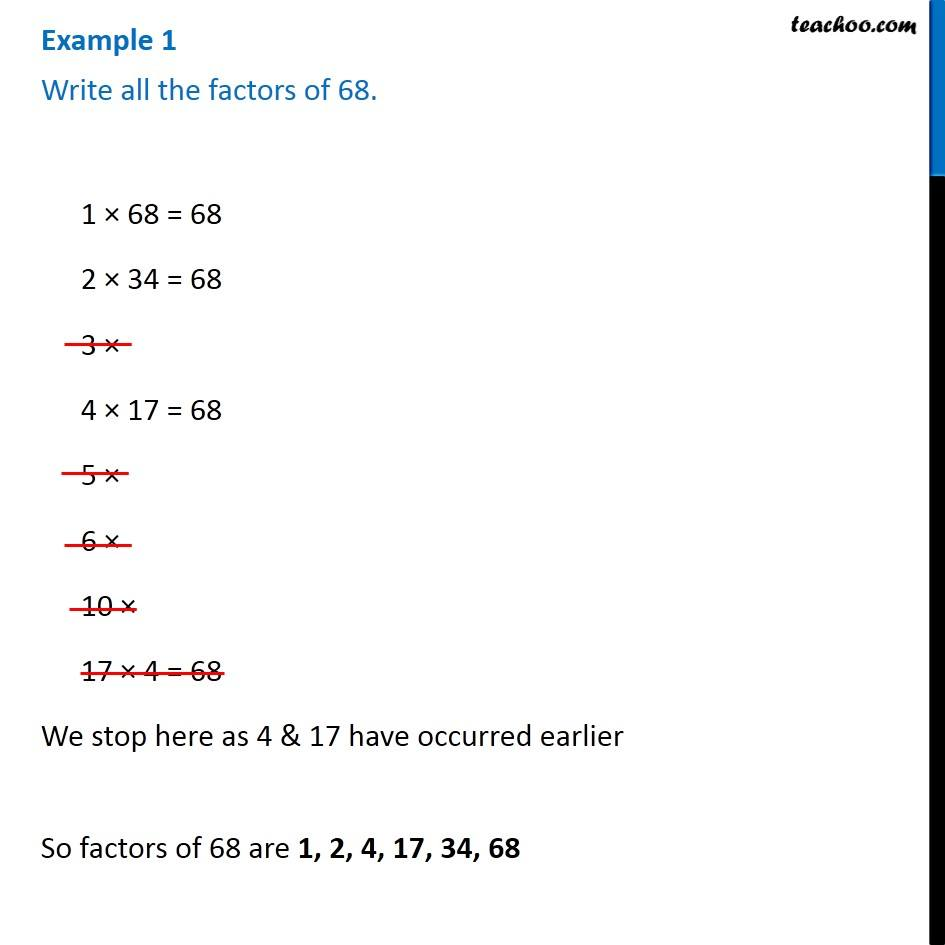1. Chapter 3 Class 6 Playing with Numbers
2. Serial order wise
3. Examples

Transcript

Example 1 Write all the factors of 68.1 × 68 = 68 2 × 34 = 68 3 × 4 × 17 = 68 5 × 6 × 10 × 17 × 4 = 68 We stop here as 4 & 17 have occurred earlier So factors of 68 are 1, 2, 4, 17, 34, 68

Examples